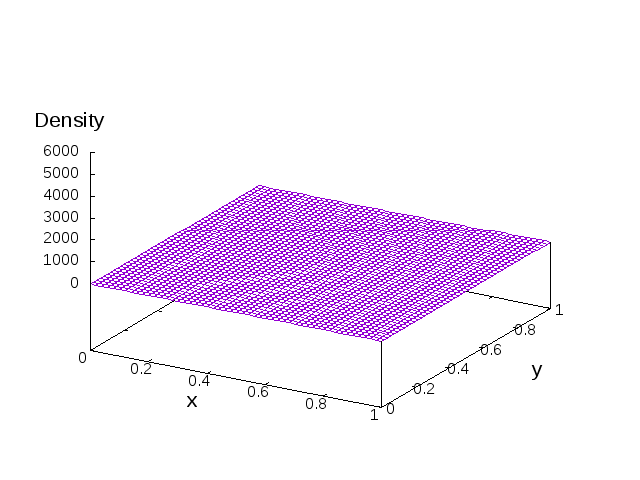# Collective motion

#### Introduction

Our research study group is interested in microorganisms that respond to extracellular changes in their environment by biased random motion towards attractants. Such movement is commonly referred to as chemo-taxis. One example would be the bacterium E.Coli. When the receptors detect a chemo-attractant gradient, the bio-motors are activated in such a way that aggregation occurs .The Keller-Segel equations can be used to model this behaviour and are given as follows$\begin{cases}\frac{\partial \rho}{\partial t}=\nabla\cdot(\nabla\rho-\rho\nabla c)\\\alpha \frac{\partial c}{\partial t} = \Delta c -c + \rho\end{cases}$

with Neumann boundary conditions, and specified initial conditions. Here$\rho(x,t)$ represents the population density and$c(x,t)$ represents the concentration of the chemoattractant.In one dimension it is known that global in time solutions exist, but in two dimensions it is possible for blow-up to occur . For the simulation below a Forward-Euler finite difference method was used where$\Delta t=10^{-6}$,$h=10^{-2}$ and$T=0.005$. The initial conditions were given by$\rho_0(x,y)=1 +(5+5\cos(2\pi(x-0.5)))(5+5\cos(2\pi(y-0.5)))$

and$c_0(x,y)=(3+3\cos(2\pi(x-0.5)))(3+3\cos(2\pi(y-0.5)))$We restricted ourselves to considering only the Parabolic-Elliptic equations (i.e.$\alpha=0$), and carried out some numerical simulations to investigate stability of solutions as well as proving our numerical scheme is convergent.$\begin{cases}\frac{\partial \rho}{\partial t}=\nabla\cdot(\nabla\rho-\rho\nabla c)\\ \Delta c =c - \rho\end{cases}$

#### References

 D. Horstmann, From 1970 until present: the Keller-Segel model in chemotaxis and its consequences, (2003)

 B. Perthame, Transport equations in biology, Springer Science & Business Media, (2006).

#### Acknowledgements

We acknowledge and thank the help of our supervisors Dr Marie-Therese Wolfram and Prof Charles Elliott.

We acknowledge the funding body EPSRC and the support from MASDOC CDT.